Activity 5 Quiz 1
identify the relationship *
1 point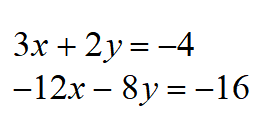Find the perpendicular slope to the slope of this line. *
1 point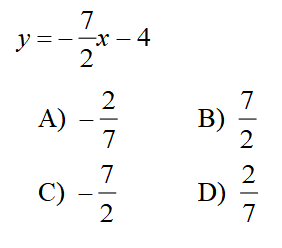Are these lines parallel? *
1 point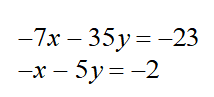identify the equations relationship *
1 point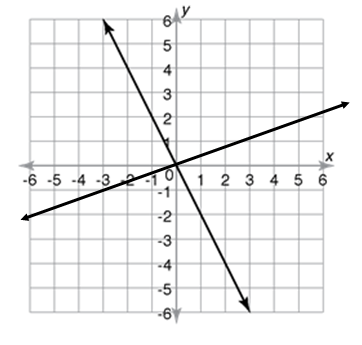find slope *
1 point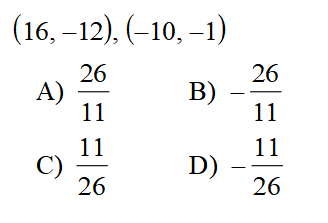identify *
1 point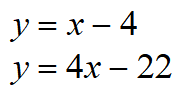Can two lines ever be both parallel and perpendicular? *
1 point
identify the equations relationship *
1 point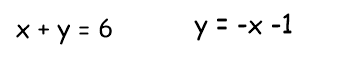Period *
Identify the line *
1 point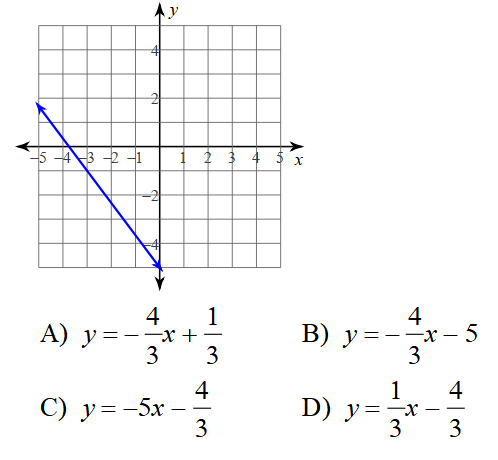Find the slope *
1 point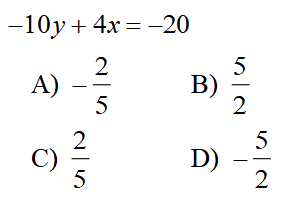Find the perpendicular slope to the slope of this line. *
1 point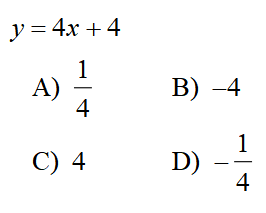identify the equations relationship *
1 point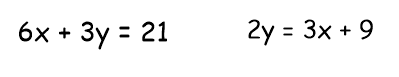What is the symbol for perpendicular? *
1 point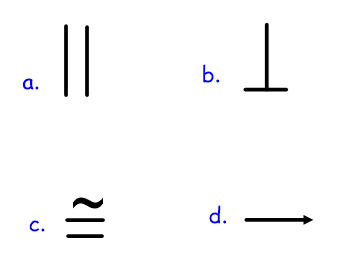Find slope *
1 point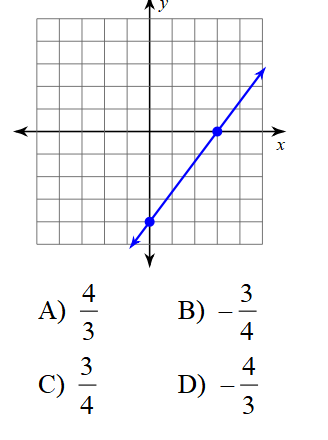Last Name *
What is the slope of a line parallel to this line? *
1 point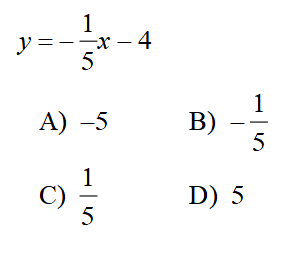Never submit passwords through Google Forms.
This content is neither created nor endorsed by Google. Report Abuse - Terms of Service - Privacy Policy